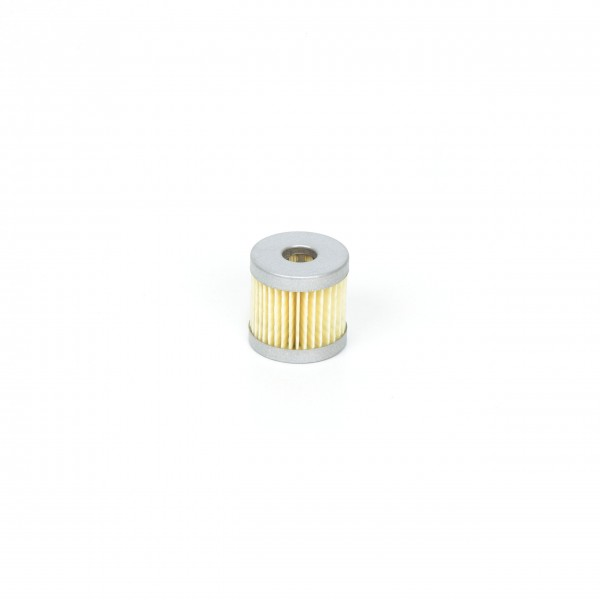# 90951800000 Filter CartridgeDelivery time: 1 - 3 business days

• 90951800000
Becker Original Filter Cartridge 90951800000 The filter medium's (paper) filtration... more
Product information "90951800000 Filter Cartridge"

Becker Original Filter Cartridge 90951800000
The filter medium's (paper) filtration efficiency is 99% at 2 µm. Filter cartridges made of paper can be cleaned by blowing through with clean dry compressed air. To ensure proper function of your Becker product, filter elements have to be cleaned and changed regularly according to the operation manual.

 Dimensions [mm]: 38-13-38 Filtration efficiency: 99% at 2 µm Use of filter cartridge: DT/VT 3.6/3.10/4.10, T 3.10-3. Filtration: Filter Cartridges Filter material: paperDT 3.10 Order number: DT3.10DT 3.10 (Index A) Order number: DT3.10-ADT 3.10 (Index B) Order number: DT3.10-BDT 3.10 (Index C) Order number: DT3.10-CDT 3.10 (Index D) Order number: DT3.10-DDT 3.10 (Index E) Order number: DT3.10-EDT 3.10 (Index F) Order number: DT3.10-FDT 3.6 Order number: DT3.6DT 3.6/08 Order number: DT3.608 DT 4.10 Order number: DT4.10DT 4.10 (single-phase AC) Order number: DT4.10single-phaseACT 3.16 DS (Index G) Order number: T3.16DS-GT 3.16 DS (Index H) Order number: T3.16DS-HT 3.16 DSK Order number: T3.16DSKT 3.16 DSK (Index A) Order number: T3.16DSK-AT 3.16 DSK (Index B) Order number: T3.16DSK-BT 3.16 DSK (Index C) Order number: T3.16DSK-CT 3.16 DSK (Index D) Order number: T3.16DSK-DT 3.16 DSK (Index E) Order number: T3.16DSK-ET 3.16 DSK (Index F) Order number: T3.16DSK-FT 3.16 DSK (Index G) Order number: T3.16DSK-GT 3.16 DSK (Index H) Order number: T3.16DSK-HT 3.25 DSK Order number: T3.25DSKT 3.25 DSK (Index A) Order number: T3.25DSK-AT 3.25 DSK (Index B) Order number: T3.25DSK-BT 3.25 DSK (Index C) Order number: T3.25DSK-CT 3.25 DSK (Index D) Order number: T3.25DSK-DT 3.25 DSK (Index E) Order number: T3.25DSK-ET 3.25 DSK (Index F) Order number: T3.25DSK-FT 3.25 DSK (Index G) Order number: T3.25DSK-GT 3.25 DSK, CSA (Index E) Order number: T3.25DSKCSA-ET 3.25 DSK, CSA (Index F) Order number: T3.25DSKCSA-FT 3.25 DSK, CSA (Index G) Order number: T3.25DSKCSA-GT 3.40 DSK Order number: T3.40DSKT 3.40 DSK (Index A) Order number: T3.40DSK-AT 3.40 DSK (Index B) Order number: T3.40DSK-BT 3.40 DSK (Index C) Order number: T3.40DSK-CT 3.40 DSK (Index D) Order number: T3.40DSK-DT 3.40 DSK (Index E) Order number: T3.40DSK-ET 3.40 DSK (Index F) Order number: T3.40DSK-FT 3.40 DSK (Index G) Order number: T3.40DSK-G T 4.10 DV Order number: T4.10DV T 4.25 DSK (Index A) Order number: T4.25DSK-A T 4.25 DSK (Index C) Order number: T4.25DSK-C T 4.25 DSK (Index X) Order number: T4.25DSK-X T 4.25 DSK (Index Z) Order number: T4.25DSK-Z T 4.40 DSK (Index A) Order number: T4.40DSK-A T 4.40 DSK (Index C) Order number: T4.40DSK-C T 4.40 DSK (Index X) Order number: T4.40DSK-X T 4.40 DSK (Index Z) Order number: T4.40DSK-ZT 4.40 DSK, /-34 (Index A) Order number: T4.40DSK-34-AT 4.40 DSK, /-34 (Index C) Order number: T4.40DSK-34-CT 4.40 DSK, /-34 (Index X) Order number: T4.40DSK-34-XT 4.40 DSK, /-34 (Index Z) Order number: T4.40DSK-34-ZVT 3.10 Order number: VT3.10VT 3.10 (Index A) Order number: VT3.10-AVT 3.10 (Index B) Order number: VT3.10-BVT 3.10 (Index C) Order number: VT3.10-CVT 3.10 (Index D) Order number: VT3.10-DVT 3.10 (Index E) Order number: VT3.10-E VT 3.6 Order number: VT3.6 VT 4.10 Order number: VT4.10
DT 4.10
Content 1 unit
VT 4.10
Content 1 unit
DT 4.16
Content 1 unit
Viewed